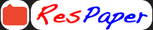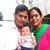Trending ▼   ResFinder# ISC Class XII Prelims 2019 : Computer Science (Johnson Grammar School (JGS) ICSE, Nacharam, Hyderabad)

6 pages, 7 questions, 0 questions with responses, 0 total responses,00Rohini Saxena +Fave Message ProfileTimelineUploads
 Home > teena_saxena >   F Also featured on: School PageFormatting page ...

JOHNSON GRAMMAR SCHOOL, ISC PRE-BOARD-2- EXAMINATION COMPUTER SCIENCE Paper 1 Theory (Candidates are allowed additional 15 minutes for only reading the paper. They must NOT start writing during this time) Class: XII Date: Marks: 70 Time: 3Hrs Answer all questions in part I (compulsory) and six Questions from Part II, choosing two questions from Section A, two questions from Section B and two questions from Section C The intended marks for questions are given in brackets { } Part I Answer all questions. While answering questions to this part, indicate briefly your working and reasoning, wherever required. Question-1 a. Convert A.B+B.C to its canonical SOP form using Boolean Algebra? b. State the dual of the following expression xy .(xy z+x+x z )and reduce after obtaining dual, using Boolean laws c. State the law represented by the following expression A+AB=A and prove it with the help of A truth table d. Verify if the following proposition is valid (a b) (a c)= a (b c) e. Convert (x +y+z ).(x+y +z)(x+y+z ).(x+y+z) into SOP form    Question 2 a) Define Computational complexity. Calculate the worst case complexity using Big O  notation for the following code segment for(int x=1;x<=a;x++) { Statements---} for(int y=1;y<=b;y++) { for(int z=1;z<=c;z++) { Statements--} } How would the complexity change if all the three loops went to N instead of a,b and c. b) Convert the following infix expression to its postfix form:A+(B-C*(D/E)*F)  c) Each element of an array X[-15 10,15 40] requires one byte of storage. If the array is  stored in column major order with the beginning location 1500, determine the location of X[5,20]. d) Differentiate between throw and throws keyword?  e) Differentiate between extends and implements keyword  Question-3 Following is a small Java code to print the first 15 numbers of the Pell series. In mathematics, the Pell numbers are an infinite sequence of integers. The sequence of Pell numbers starts with 1, and then each Pell number is the sum of twice the previous Pell number and the Pell number before that.: thus, 70 is the companion to 29, and 70 = 2 29 + 12 = 58 + 12. The first few terms of the sequence are : 1, 2, 5, 12, 29, 70, 169, 408, 985, 2378, 5741, 13860,

Related ResPapers
 ISC Class XII Prelims 2021 : Computer Science (Father Leblond School, Darjeeling) by rudra199140 ISC Class XII Prelims 2020 : Computer Science (Don Bosco School, Liluah, Howrah) : Paper - 1(Theory) by mayukh_mondal ISC Class XII Prelims 2023 : Computer Science (St. Johns School, Ghazipur) by 123456_anand ISC Class XII Prelims 2019 : Computer Science (Jamnabai Narsee School (JNS), Mumbai) by minujoshiFormatting page ...Formatting page ...Formatting page ...Formatting page ...Formatting page ...